# RD Sharma Class 8 Solutions Chapter 7 Factorizations Ex 7.7

## RD Sharma Class 8 Solutions Chapter 7 Factorizations Ex 7.7

These Solutions are part of RD Sharma Class 8 Solutions. Here we have given RD Sharma Class 8 Solutions Chapter 7 Factorizations Ex 7.7

Other Exercises

Factorize each of the following algebraic expressions :
Question 1.
x2 + 12x – 45
Solution: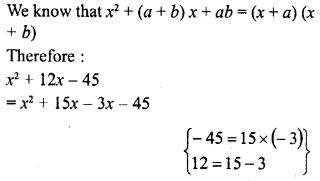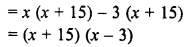Question 2.
40 + 3x – x2
Solution: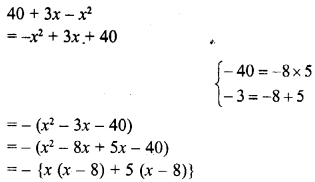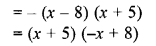Question 3.
a2 + 3a-88
Solution: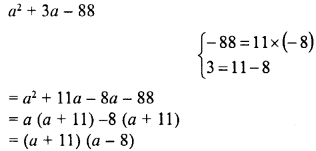Question 4.
a2 – 14a – 51
Solution: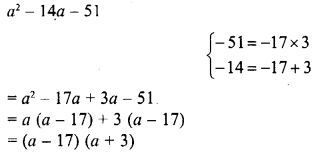Question 5.
x2 + 14x + 45
Solution: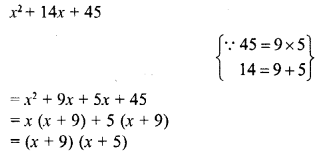Question 6.
x2 – 22x + 120
Solution:Question 7.
x2– 11x – 42
Solution: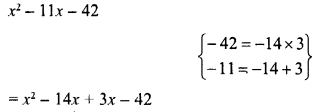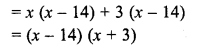Question 8.
a2 + 2a – 3
Solution: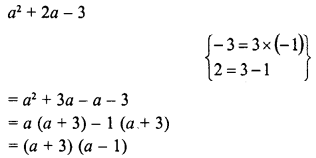Question 9.
a2 + 14a + 48
Solution: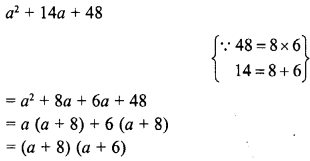Question 10.
x2 – 4x – 21
Solution: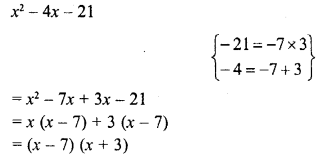Question 11.
y2 – 5y-36
Solution: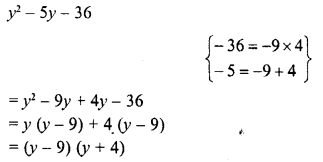Question 12.
(a2-5a)2-36
Solution: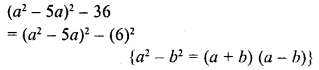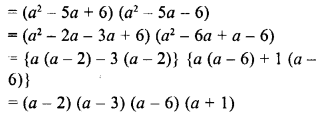Question 13.
(a + 7) (a – 10) + 16
Solution: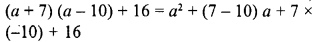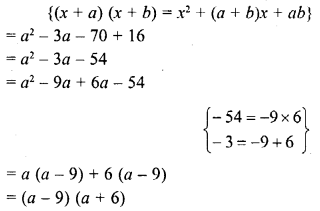Hope given RD Sharma Class 8 Solutions Chapter 7 Factorizations Ex 7.7 are helpful to complete your math homework.

If you have any doubts, please comment below. Learn Insta try to provide online math tutoring for you.